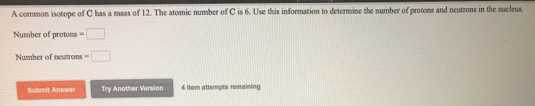# Problem: A common of C has a mass of 12. The atomic number of C is 6. Use this information to determine the number of protons and neutrons in the nucleus. Number of protons = Number of neutrons =

###### FREE Expert Solution

Atomic number tells the number of protons. If it has 6 as its atomic number, its # of proton is 6 as well.

95% (403 ratings)###### Problem Details

A common of C has a mass of 12. The atomic number of C is 6. Use this information to determine the number of protons and neutrons in the nucleus.

Number of protons =

Number of neutrons =